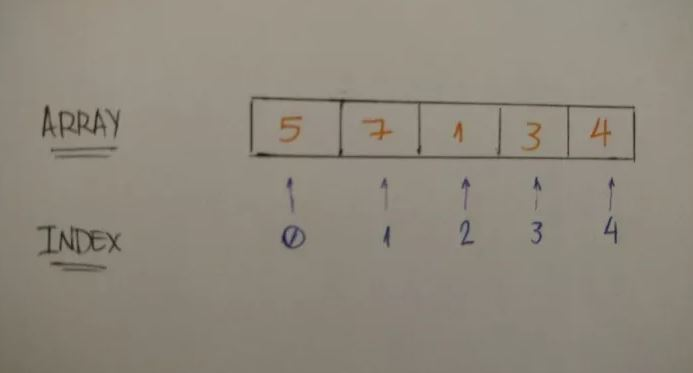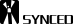# 从Zero到Hero，一文掌握Python关键代码

2018年4月1日09:33:53 4 370 views

Python 是一个高层次的结合了解释性、编译性、互动性和面向对象的脚本语言。Python 的设计具有很强的可读性，相比其他语言经常使用英文关键字，其他语言的一些标点符号，它具有比其他语言更有特色语法结构。

`one = 1`

```two = 2
some_number = 10000```

```# booleans
true_boolean = True
false_boolean = False
# string
my_name = "Leandro Tk"
# float
book_price = 15.80```

「If」语句通过表达式评估一个语句是真或假。如果是真，则向下执行「If」条件中的子语句。比如：

```if True:
print("Hello Python If")
if 2 > 1:
print("2 is greater than 1")```

2 比 1 大，因此「print」代码被执行。如果「If」表达式是假的，则「else」下的子语句将被执行。

```if 1 > 2:
print("1 is greater than 2")
else:
print("1 is not greater than 2")```

```if 1 > 2:
print("1 is greater than 2")
elif 2 > 1:
print("1 is not greater than 2")
else:
print("1 is equal to 2")```

While 循环：当该语句为真，以下代码将被执行，并打印从 1 到 10 的数字。

```num = 1
while num <= 10:
print(num)
num += 1```

While 循环需要一个「循环条件」。如果它为真，则继续迭代。在以上实例中，当 num 为 11，则循环条件为假，我们结束循环。

```loop_condition = True
while loop_condition:
print("Loop Condition keeps: %s" %(loop_condition))
loop_condition = False```

```for i in range(1, 11):
print(i)
```

`my_integers = [1, 2, 3, 4, 5]````my_integers = [5, 7, 1, 3, 4]
print(my_integers) # 5
print(my_integers) # 7
print(my_integers) # 4```

```relatives_names = [
"Toshiaki",
"Juliana",
"Yuji",
"Bruno",
"Kaio"
]
print(relatives_names) # Kaio```

```bookshelf = []
bookshelf.append("The Effective Engineer")
bookshelf.append("The 4 Hour Work Week")
print(bookshelf) # The Effective Engineer
print(bookshelf) # The 4 Hour Work Week```

append 方法非常简单，我们只需要对需要添加的元素应用该方法就能将其添加到列表的末尾。

```dictionary_example = {
"key1": "value1",
"key2": "value2",
"key3": "value3"
}```

```dictionary_tk = {
"name": "Leandro",
"nickname": "Tk",
"nationality": "Brazilian"
}
print("My name is %s" %(dictionary_tk["name"])) # My name is Leandro
print("But you can call me %s" %(dictionary_tk["nickname"])) # But you can call me Tk
print("And by the way I'm %s" %(dictionary_tk["nationality"])) # And by the way I'm Brazilian```

```dictionary_tk = {
"name": "Leandro",
"nickname": "Tk",
"nationality": "Brazilian",
"age": 24
}
print("My name is %s" %(dictionary_tk["name"])) # My name is Leandro
print("But you can call me %s" %(dictionary_tk["nickname"])) # But you can call me Tk
print("And by the way I'm %i and %s" %(dictionary_tk["age"], dictionary_tk["nationality"])) # And by the way I'm Brazilian```

```dictionary_tk = {
"name": "Leandro",
"nickname": "Tk",
"nationality": "Brazilian"
}
dictionary_tk['age'] = 24
print(dictionary_tk) # {'nationality': 'Brazilian', 'age': 24, 'nickname': 'Tk', 'name': 'Leandro'}```

```bookshelf = [
"The Effective Engineer",
"The 4 hours work week",
"Zero to One",
"Lean Startup",
"Hooked"
]
for book in bookshelf:
print(book)```

```dictionary = { "some_key": "some_value" }
for key in dictionary:
print("%s --> %s" %(key, dictionary[key]))
# some_key --> some_value```

```dictionary = { "some_key": "some_value" }
for key, value in dictionary.items():
print("%s --> %s" %(key, value))
# some_key --> some_value```

```dictionary_tk = {
"name": "Leandro",
"nickname": "Tk",
"nationality": "Brazilian",
"age": 24
}
for attribute, value in dictionary_tk.items():
print("My %s is %s" %(attribute, value))
# My name is Leandro
# My nickname is Tk
# My nationality is Brazilian
# My age is 24```

Python 是一种面向对象的程序语言，因此它也有类（Class）与对象（Object）这两个概念。在了解 Python 面向对象编程的案例前，我们需要先熟悉面向对象编程的一些基本概念：

• 类 (Class)：用来描述具有相同的属性和方法的对象的集合。它定义了该集合中每个对象所共有的属性和方法。对象是类的实例。
• 类变量：类变量在整个实例化的对象中是公用的。类变量定义在类中且在函数体之外。类变量通常不作为实例变量使用。
• 数据成员：类变量或者实例变量用于处理类及其实例对象的相关的数据。
• 方法重写：如果从父类继承的方法不能满足子类的需求，可以对其进行改写，这个过程叫方法的覆盖（override），也称为方法的重写。
• 实例变量：定义在方法中的变量，只作用于当前实例的类。
• 继承：即一个派生类（derived class）继承基类（base class）的字段和方法。继承也允许把一个派生类的对象作为一个基类对象对待。例如，一个「狗」类的对象派生自「动物」类，这是模拟"是一个（is-a）"关系（狗是一种动物）。
• 实例化：创建一个类的实例，类的具体对象。
• 方法：类中定义的函数。
• 对象：通过类定义的数据结构实例。对象包括两个数据成员（类变量和实例变量）和方法。

```class Vehicle:
pass```

```car = Vehicle()
print(car) # <__main__.Vehicle instance at 0x7fb1de6c2638>```

```class Vehicle:
def __init__(self, number_of_wheels, type_of_tank, seating_capacity, maximum_velocity):
self.number_of_wheels = number_of_wheels
self.type_of_tank = type_of_tank
self.seating_capacity = seating_capacity
self.maximum_velocity = maximum_velocity```

__init__() 方法是一种特殊的方法，被称为类的构造函数或初始化方法，当创建 vehicle 类的实例时就会调用该方法来定义这些属性。若我们希望能创建 Tesla Model S 这一个对象，根据其有四个车轮、电力驱动、四座容量和最大时速为 250km/hour 的特征，我们能创建对象：

`tesla_model_s = Vehicle(4, 'electric', 5, 250)`

```class Vehicle:
def __init__(self, number_of_wheels, type_of_tank, seating_capacity, maximum_velocity):
self.number_of_wheels = number_of_wheels
self.type_of_tank = type_of_tank
self.seating_capacity = seating_capacity
self.maximum_velocity = maximum_velocity
def number_of_wheels(self):
return self.number_of_wheels
def set_number_of_wheels(self, number):
self.number_of_wheels = number```

```class Vehicle:
def __init__(self, number_of_wheels, type_of_tank, seating_capacity, maximum_velocity):
self.number_of_wheels = number_of_wheels
self.type_of_tank = type_of_tank
self.seating_capacity = seating_capacity
self.maximum_velocity = maximum_velocity
@property
def number_of_wheels(self):
return self.number_of_wheels
@number_of_wheels.setter
def number_of_wheels(self, number):
self.number_of_wheels = number```

```tesla_model_s = Vehicle(4, 'electric', 5, 250)
print(tesla_model_s.number_of_wheels) # 4
tesla_model_s.number_of_wheels = 2 # setting number of wheels to 2
print(tesla_model_s.number_of_wheels) # 2```

```class Vehicle:
def __init__(self, number_of_wheels, type_of_tank, seating_capacity, maximum_velocity):
self.number_of_wheels = number_of_wheels
self.type_of_tank = type_of_tank
self.seating_capacity = seating_capacity
self.maximum_velocity = maximum_velocity
def make_noise(self):
print('VRUUUUUUUM')```

```tesla_model_s = Vehicle(4, 'electric', 5, 250)
tesla_model_s.make_noise() # VRUUUUUUUM```

```class Person:
def __init__(self, first_name):
self.first_name = first_name```

```tk = Person('TK')
print(tk.first_name) # => TK```

```class Person:
first_name = 'TK'```

```tk = Person()
print(tk.first_name) # => TK```

```tk = Person('TK')
tk.first_name = 'Kaio'
print(tk.first_name) # => Kaio```

```class Person:
def __init__(self, first_name, email):
self.first_name = first_name
self._email = email```

```tk = Person('TK', 'tk@mail.com')
print(tk._email) # tk@mail.com```

```class Person:
def __init__(self, first_name, email):
self.first_name = first_name
self._email = email
def update_email(self, new_email):
self._email = new_email
def email(self):
return self._email```

```tk = Person('TK', 'tk@mail.com')
print(tk.email()) # => tk@mail.com
tk._email = 'new_tk@mail.com'
print(tk.email()) # => tk@mail.com
tk.update_email('new_tk@mail.com')
print(tk.email()) # => new_tk@mail.com```

```class Person:
def __init__(self, first_name, age):
self.first_name = first_name
self._age = age
def show_age(self):
return self._age```

```tk = Person('TK', 25)
print(tk.show_age()) # => 25```

```class Person:
def __init__(self, first_name, age):
self.first_name = first_name
self._age = age
def _show_age(self):
return self._age```

```tk = Person('TK', 25)
print(tk._show_age()) # => 25```

```class Person:
def __init__(self, first_name, age):
self.first_name = first_name
self._age = age
def show_age(self):
return self._get_age()
def _get_age(self):
return self._age
tk = Person('TK', 25)
print(tk.show_age()) # => 25```

```class Car:
def __init__(self, number_of_wheels, seating_capacity, maximum_velocity):
self.number_of_wheels = number_of_wheels
self.seating_capacity = seating_capacity
self.maximum_velocity = maximum_velocity```

```my_car = Car(4, 5, 250)
print(my_car.number_of_wheels)
print(my_car.seating_capacity)
print(my_car.maximum_velocity)```

```class ElectricCar(Car):
def __init__(self, number_of_wheels, seating_capacity, maximum_velocity):
Car.__init__(self, number_of_wheels, seating_capacity, maximum_velocity)```

```my_electric_car = ElectricCar(4, 5, 250)
print(my_electric_car.number_of_wheels) # => 4
print(my_electric_car.seating_capacity) # => 5
print(my_electric_car.maximum_velocity) # => 250```

Beautiful.## 目前评论：4   其中：访客  3   博主  1

•Black-Feather 0

嗯值得学习~

•我是小马 1

简单易懂，适合初学者。

•51changxue Admin

@我是小马 你好，很高兴能帮到你。

•听风梦 0

简单易懂，有个漫画就更好了